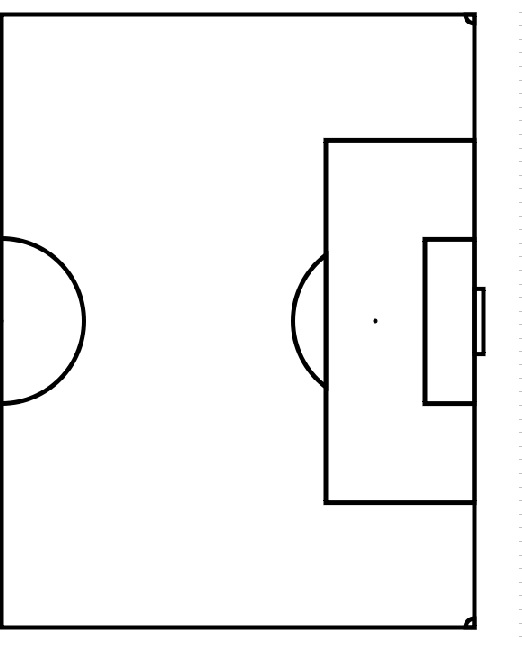This Shows the speed of a soccer free kick and the Goalie to show how fast a keeper has to travel from post to post in order to block the ball.

This document requires an HTML5-compliant browser.
 b 0 0 2.388 c 0.01 0 0.45

In order to score from a distance of 23.8 yards out from the goal box, you need to know how hard to hit the ball. The average time it takes for a free kick to get from the its place of rest to the goal is 4 seconds.

First we will find the velocity of the ball.
Velocity = Distance / Time
V= 23.8/4
V= 5.95
Second to find the Force you need to hit the balll with you use Newtons Second Law of Motion
Force= (Ball weight x Velocity)/ The time of contact between a player’s shoe and the ball
Ball Weight = .45kg
Velocity= 5.95 yrds/sec
Time of Contact from players shoe to the ball= .05 seconds
F= (.45 x 5.95)/.05
F= 53.55 Newtons

The Goalie has to travel 3.9 yards in 3 seconds because the wall blocks his view of the ball for 1 second

S = 3.9/3
S = 1.3 yds/sec

This means that the minimum speed the keeper can run from 1st post to second post is 1.3 yds/sec in order to block the ball.

Picture was created in paint by me.

App generated by Geometry Expressions